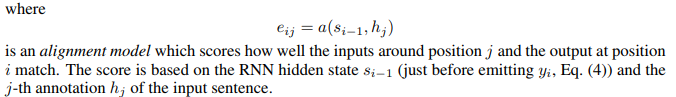# Attention weights implementation in the official tutorial is inconsistent with the listed publications

This image is from the tutorial at https://pytorch.org/tutorials/intermediate/seq2seq_translation_tutorial.html#sphx-glr-intermediate-seq2seq-translation-tutorial-py.

When deriving the attention weights, the input of “attn” is the concatenation of previous decoder hidden state and current decoder input, output is the attention weights who will be applied to the encoder outputs. The shape of this output is (batch_size, FIXED_length_of_encoder_input, 1)

BUT according to “Neural Machine Translation by Jointly Learning to Align and Translate”, when deriving attention weights we should use the concatenation of previous decoder hidden state and each of the encoder outputs to get the corresponding energy e_ij. Output size is (batch_size, ACTUAL_length_of_encoder_input, 1)

Could anyone justify this offical tutorial implementation? (I mean using the decoder input in deriving attention weights) A reference paper also helps.

There is another question:
In every forward function of the tutorial, it reshapes the embedding:
embedded = self.embedding(input).view(1, 1, -1)
Why is that?

Where do you get the fixed length from?

On first sight, it looks like the training is done with a minibatch size of 1 and so the input_length in the train function’s for loop `for ei in range(input_length):` is just the length of the training input.

Best regards

Thomas

In class AttnDecoderRNN, you can find:
def init(self, hidden_size, output_size, dropout_p=0.1, max_length=MAX_LENGTH):
self.max_length = max_length
self.attn = nn.Linear(self.hidden_size * 2, self.max_length)
So the sequence length of attention weights is determined by the neural network architecture.
The time complexity of the attention weights computations in the tutorial is:
length_of_target_sequence * 1We should compare each of the previous decoder hidden states at every time stamp to each of the encoder outputs at every time stamp. The time complexity is:
length_of_target_sequence * length_of_input_sequence

Please notice the time complexity is reduced from n square to n. Could anyone give me the reference paper of this implementation in the tutorial. Using the decoder input and previous decoder hidden state to predict the attention weights does not make too much sense to me.

I think that that is just a simplification in the implementation. The blue box note below the AttnDecoderRNN alludes to the fact that this approach is more limited than others.
What happens with the overly long attention weights is that they will get multiplied by the 0s in `decoder_outputs`.
Having the previous state and decoder input to predict weights seems not too uncommon to me - it sounds vaguely similar to what I have seen elsewhere. (I like to advocate Graves’ Handwriting RNN for a gentle first contact with attention.)
I don’t think the computational complexity is the main limitation here.

Best regards

Thomas

1 Like